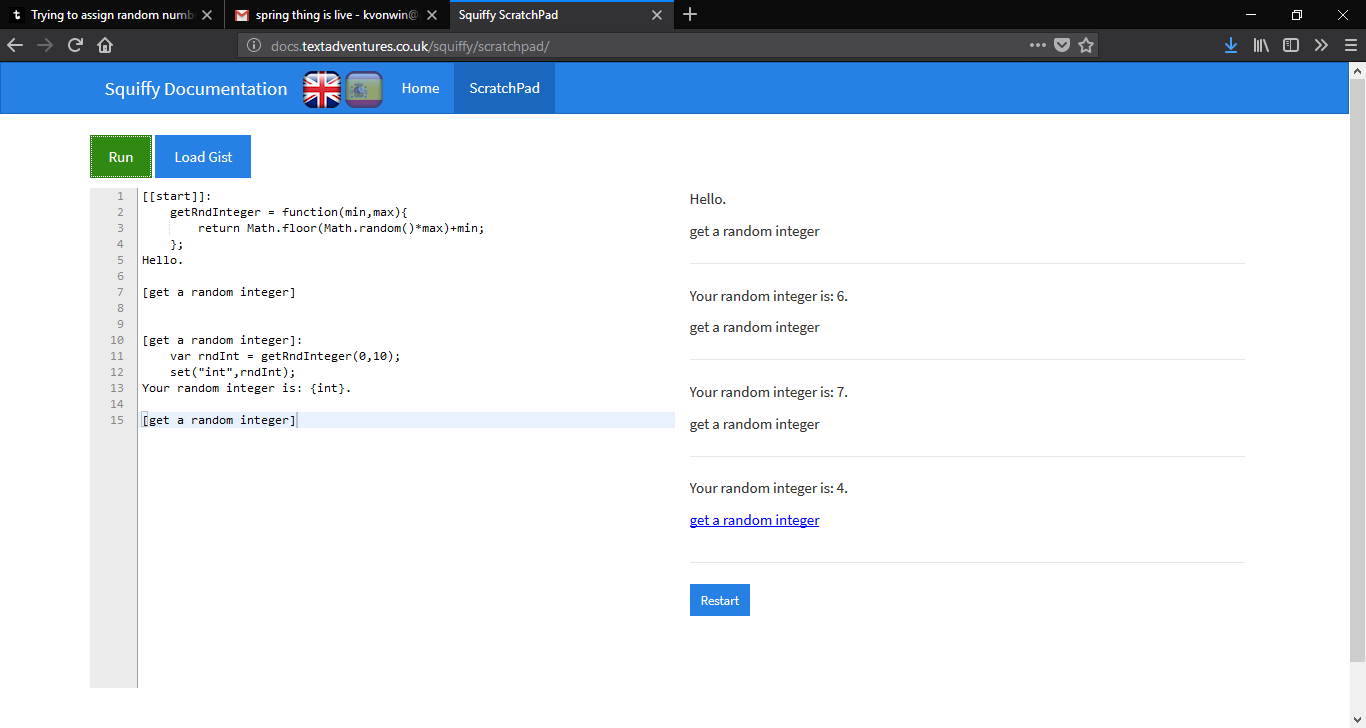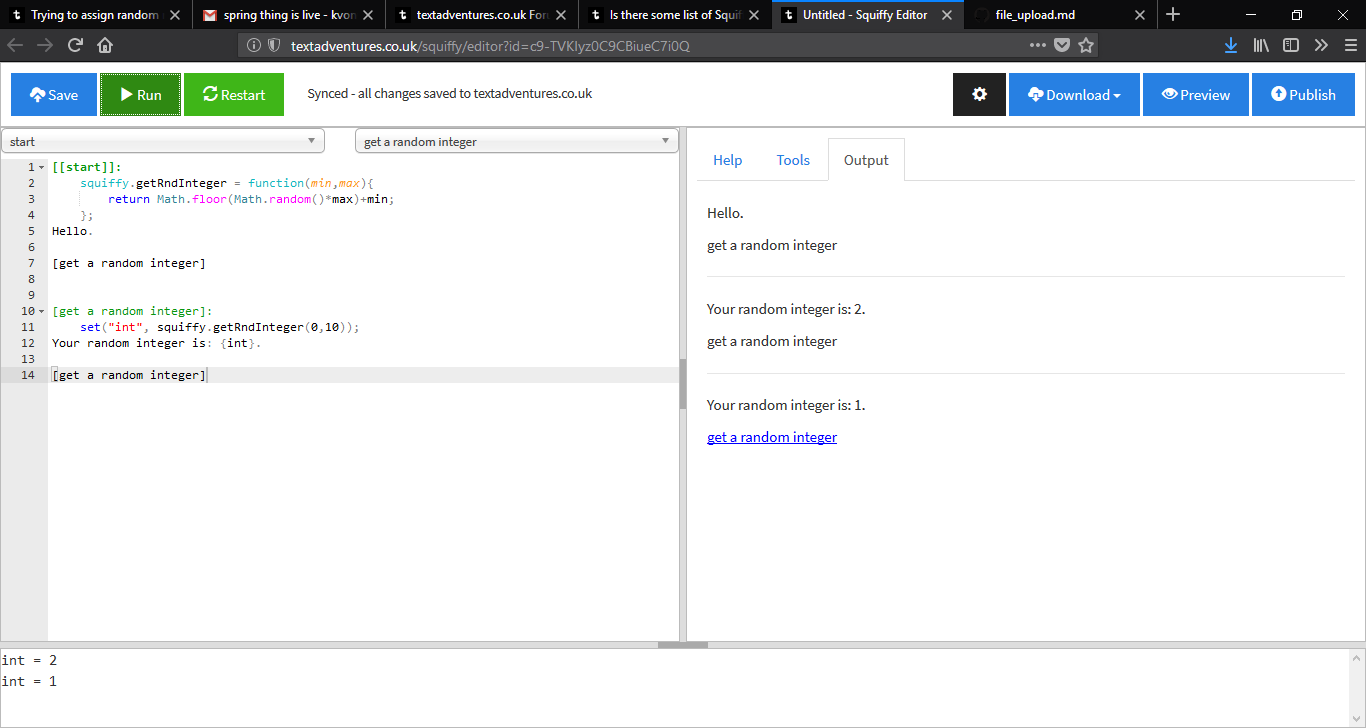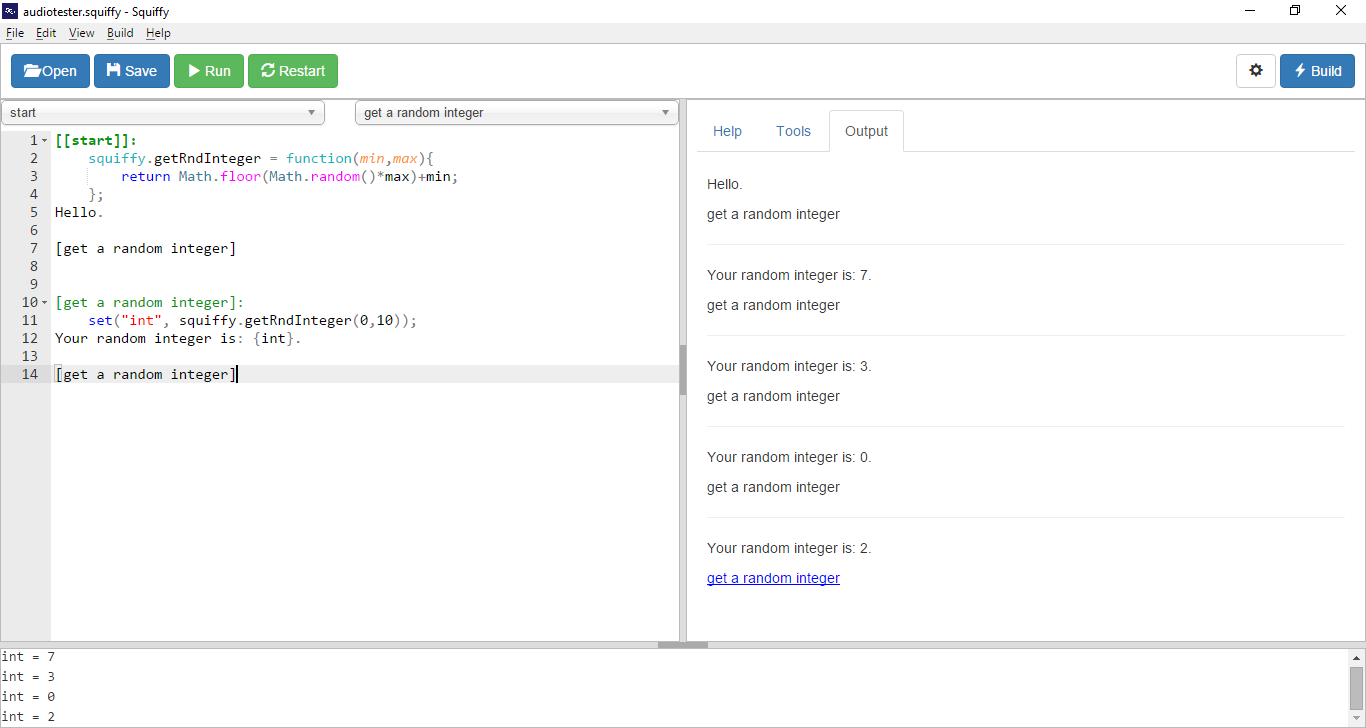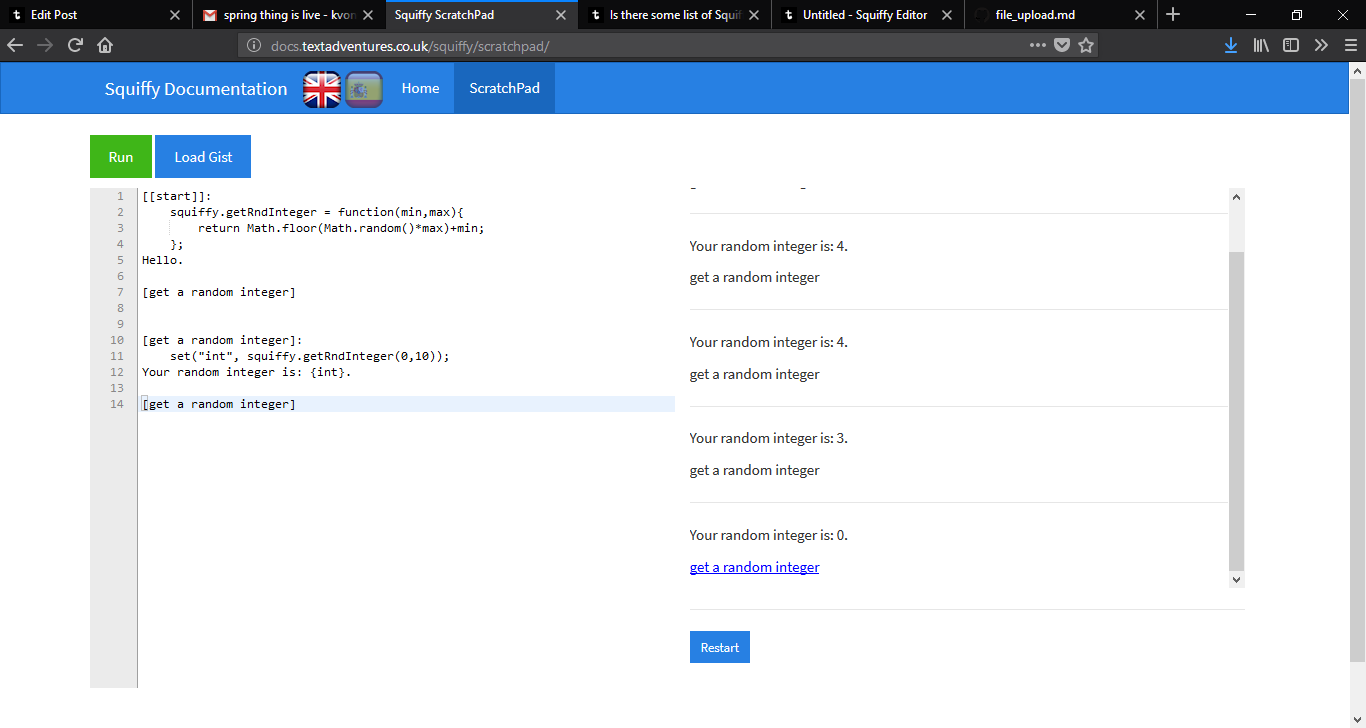## Trying to assign random number to a variable/attributeI'm very very new to programming and Javascript. I have a problem.

I'm writing a story in which time will pass with each player turn and certain events will occur if a particular random number is "rolled" at various points in the story.

So I am at the point now where I am just starting to play with this idea. I know that I can have a master section that can increment an attribute that represents passage of time (or amount of player clicks between sections, basically). My thought is that I want to generate a random number at certain points and assign it to a variable so that I can test it in if/then statements.

I took a look online and see that I can generate a random number in a specified range using a Javascript function:

``````            function getRndInteger(min, max) {
return Math.floor(Math.random() * (max - min + 1) ) + min;
}
``````

My thought was that, since a Master section runs every turn, it would be very inefficient to put the function in a Master section. So I just put the function getRndInteger at the top of my squiffy file, right after @title and @start and before any sections or passages.

Then, to test it out, I put the following code in one of my sections:

``````    var result = getRndInteger(1, 10)

result is: {result}
``````

But I'm not getting my desired outcome. The call doesn't seem to be working. It doesn't work if I put the function in a master section either.

Is my thought about keeping the function out of a master section to increase efficiency correct? Why can't I call the function, get a number, and assign it to variable/attribute "result"? Are Javascript variables and Squiffy attributes one and the same? Are any of my other assumptions incorrect or misguided?What numbers are you getting??? are they outside the 1 to 10 range?
An easy way to test this is to call the GetRndInteger several times in a roll and watch the results.
In Quest, GetRndInteger is a function that you do not need to create...K.V.

Try this (changing 0 and 10 to whatever you please):

``````[[start]]:
getRndInteger = function(min,max){
return Math.floor(Math.random()*max)+min;
};
Hello.

[get a random integer]

[get a random integer]:
var rndInt = getRndInteger(0,10);
set("int",rndInt);

[get a random integer]
``````Awesome! Thank you K.V.; I will try that ASAP.K.V., unfortunately your code doesn't seem to work as a standalone game or in my game. A blank screen is shown when the game is run.

My code, it "gets past" the first part but calling the function doesn't work: it returns null.Update--It's late and I'm tired but I can only seem to get it to work like this:

``````[[This-is-a-section]]

[[This-is-a-section]]:
function getRndInteger(min, max) {
return Math.floor(Math.random() * (max - min + 1) ) + min;
};

set("result",getRndInteger(1, 10))

result is: {result}
``````

However, I can't then put:

``````        set("result2",getRndInteger(1, 10))

result is: {result2}
``````

in a passage within the same section and get a 2nd variable.

I can only get it to work if I repeat the function in every section or passage I want to get a random number in, as above.

How can I define the function in one section and call it in a different passage?K.V.

Really? Is that in a browser or in a build of Squiffy?

I was doing that in Scratchpad:...but that does not work elsewhere.

This seems to work quite well everywhere, though:

``````[[start]]:
squiffy.getRndInteger = function(min,max){
return Math.floor(Math.random()*max)+min;
};
Hello.

[get a random integer]

[get a random integer]:
set("int", squiffy.getRndInteger(0,10));

[get a random integer]
``````That does it! In your example, the function is defined in the section and then called in a sub-passage, and if that was as far as it would go, I would have been happy with that, but I tested it and you can also define the function in one section and call it in a completely different section and it still works. So that's very sweet! ;)

Like this (just adding extra brackets around your passage to make it a separate section):

``````[[start]]:
squiffy.getRndInteger = function(min,max){
return Math.floor(Math.random()*max)+min;
};
Hello.

[[get a random integer]]

[[get a random integer]]:
set("int", squiffy.getRndInteger(0,10));

[[get a random integer]]
``````

Thanks K.V.!

This topic is now closed. Topics are closed after 60 days of inactivity.

Quest
Squiffy
ActiveLit

Documentation
Blog
GitHub

Forums